/

### The binomial distribution

The binomial distribution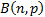is the discrete probability distribution applicable to the number of successes in a sequence ofindependent yes/no experiments each of which has a success probability of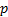. Each individual success/failure experiment is called a Bernoulli trial, so if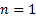then the binomial distribution is a Bernoulli distribution.

It has the following characteristics:

 Distribution name Binomial distribution Common notation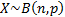Parameters= number of (independent) trials, positive integer= probability of success in each trial,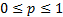Support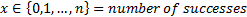Probability mass function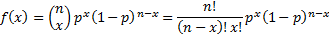Cumulative distribution function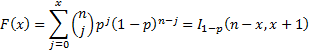Mean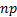Variance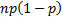Skewness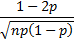(Excess) kurtosis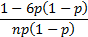Characteristic function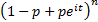Other comments The Bernoulli distribution is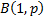and corresponds to the likelihood of success of a single experiment.  Its probability mass function and cumulative distribution function are: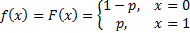The Bernoulli distribution with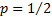, i.e.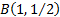, has the minimum possible excess kurtosis, i.e.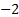.   The mode ofis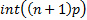if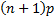is 0 or not an integer and isif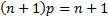. If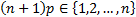then the distribution is bi-modal, with modesand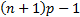.

The binomial distribution is often used to model the number of successes in a sample size offrom a population size of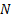. Since such samples are not independent, the resulting distribution is actually a hypergeometric distribution and not a binomial distribution. However if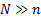then the binomial distribution becomes a good approximation to the relevant hypergeometric distribution and is thus often used.

In the above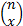is the binomial coefficient.

Nematrian web functions

Functions relating to the above distribution may be accessed via the Nematrian web function library by using a DistributionName of “binomial”. For details of other supported probability distributions see here.